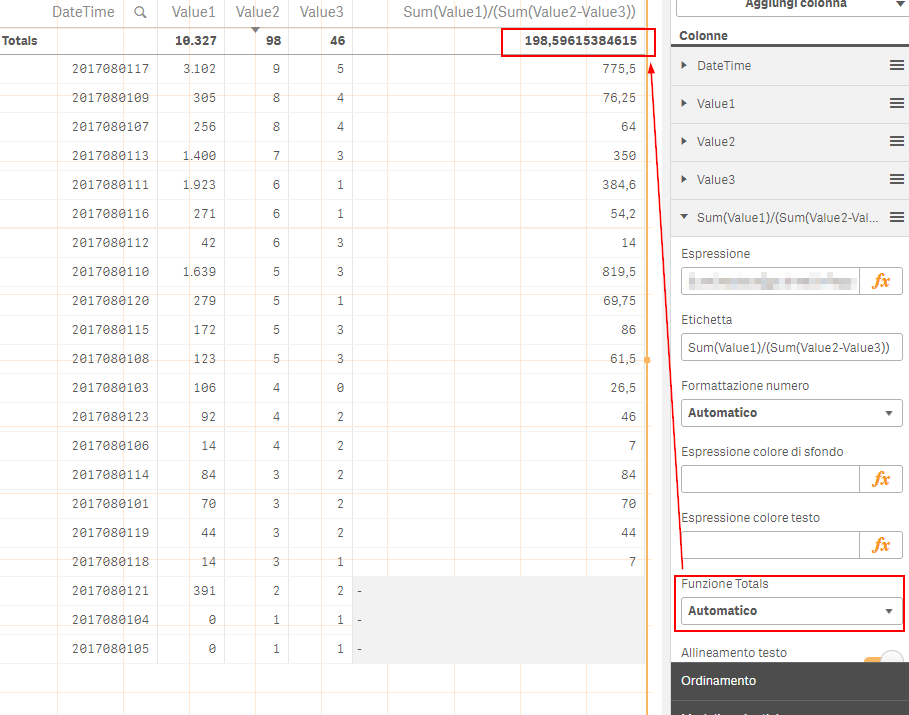# Qlik Sense App Development

Discussion board where members can learn more about Qlik Sense App Development and Usage.

Announcements
Qlik® Product Spotlight: Discover what’s possible. Get more from our products.
See for yourself. Register today.
Valued Contributor

## Straight Table: Automatic Totals Function doesn't works as expected

Hi Experts,

i have one question regarding the "Total" function on Straight Table.

As you can see, i've set the total function on "Automatic" mode and i would like to know which are the calculations QlikSense Server (v 3.0.2) is doing to calculate the total 198,59615384615 because this number is not a sum(total), avg, count of the numbers below so... what calculations is it doing ?

Yes, i know that 10.327/ (98-46)=198,59615384615 but i would expect that the TOTAL function (set on automatic mode) returns the sum.

Which type of reasoning is doing QlikSense by using "Automatic" total function ?

I've attached the data i'm using.1 Solution

Accepted Solutions
Honored Contributor III

## Re: Straight Table: Automatic Totals Function doesn't works as expected

When in Auto Mode, It calculates your expression on Overall Level considering total of each parameters in Expression.

When Total is set to Sum, it will sum up values of Rows.

3 Replies
Honored Contributor III

## Re: Straight Table: Automatic Totals Function doesn't works as expected

When in Auto Mode, It calculates your expression on Overall Level considering total of each parameters in Expression.

When Total is set to Sum, it will sum up values of Rows.

Valued Contributor